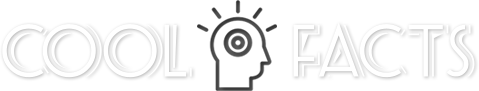NextPrevious# Why are truth tables important to computers?

Mathematical and Formal Logic Read more from
Chapter Foundations of Mathematics

In many ways, truth tables are directly connected to digital logic circuits; in the case of computers, the terms would be AND, OR, NAND, NOR, NOT, XOR, or the “gates” that open and close in response to such terms. In such a circuit, values at each point can take on values of only true (1) or false (0); this is also known as the computer binary system. In general, there is also a three-valued logic, in which possible values are true, false, and “undecided.” A further generalization called fuzzy logic examines the “truth” as a continuous quantity ranging from 0 to 1. (For more about fuzzy logic and computers, see “Math in Computing.”)

Close

This is a web preview of the "The Handy Math Answer Book" app. Many features only work on your mobile device. If you like what you see, we hope you will consider buying. Get the App